# Chapter 25 Many models

Author: Ron Reviewer:

## 25.1 Introduction

Note:

1. Use many simple models to better understand complex datasets
2. Use list-columns to store arbitrary data stracture in a data frame
3. Use broom package to turn models into tidy data

### 25.1.1 Prerequisites

``````library(modelr)
library(tidyverse)``````
``````## Loading tidyverse: ggplot2
``## Conflicts with tidy packages ----------------------------------------------``
``````## filter(): dplyr, stats
## lag():    dplyr, stats``````
``library(gapminder)``

## 25.2 gapminder

``gapminder``
``````## # A tibble: 1,704 x 6
##        country continent  year lifeExp      pop gdpPercap
##         <fctr>    <fctr> <int>   <dbl>    <int>     <dbl>
##  1 Afghanistan      Asia  1952  28.801  8425333  779.4453
##  2 Afghanistan      Asia  1957  30.332  9240934  820.8530
##  3 Afghanistan      Asia  1962  31.997 10267083  853.1007
##  4 Afghanistan      Asia  1967  34.020 11537966  836.1971
##  5 Afghanistan      Asia  1972  36.088 13079460  739.9811
##  6 Afghanistan      Asia  1977  38.438 14880372  786.1134
##  7 Afghanistan      Asia  1982  39.854 12881816  978.0114
##  8 Afghanistan      Asia  1987  40.822 13867957  852.3959
##  9 Afghanistan      Asia  1992  41.674 16317921  649.3414
## 10 Afghanistan      Asia  1997  41.763 22227415  635.3414
## # ... with 1,694 more rows``````

### 25.2.1 Nested data

use `nest()` function to create a dataframe of dataframes which has a column called `data`.

``````by_country <- gapminder %>%
group_by(country, continent) %>%
nest()``````

Use `mutate` and `purrr::map` to generate new column, the model itself, which is an S3 object.

``````country_model <- function(df) {
lm(lifeExp ~ year, data = df)
}
by_country <- by_country %>%
mutate(model = map(data, country_model))
by_country``````
``````## # A tibble: 142 x 4
##        country continent              data    model
##         <fctr>    <fctr>            <list>   <list>
##  1 Afghanistan      Asia <tibble [12 x 4]> <S3: lm>
##  2     Albania    Europe <tibble [12 x 4]> <S3: lm>
##  3     Algeria    Africa <tibble [12 x 4]> <S3: lm>
##  4      Angola    Africa <tibble [12 x 4]> <S3: lm>
##  5   Argentina  Americas <tibble [12 x 4]> <S3: lm>
##  6   Australia   Oceania <tibble [12 x 4]> <S3: lm>
##  7     Austria    Europe <tibble [12 x 4]> <S3: lm>
##  8     Bahrain      Asia <tibble [12 x 4]> <S3: lm>
##  9  Bangladesh      Asia <tibble [12 x 4]> <S3: lm>
## 10     Belgium    Europe <tibble [12 x 4]> <S3: lm>
## # ... with 132 more rows``````

### 25.2.2 Unnesting

`purrr::map2` can map mulitple inputs simultaneously

``````by_country <- by_country %>%
# data here is the column name, so is model.
)

unnest(by_country,resids)``````
``````## # A tibble: 1,704 x 7
##        country continent  year lifeExp      pop gdpPercap       resid
##         <fctr>    <fctr> <int>   <dbl>    <int>     <dbl>       <dbl>
##  1 Afghanistan      Asia  1952  28.801  8425333  779.4453 -1.10629487
##  2 Afghanistan      Asia  1957  30.332  9240934  820.8530 -0.95193823
##  3 Afghanistan      Asia  1962  31.997 10267083  853.1007 -0.66358159
##  4 Afghanistan      Asia  1967  34.020 11537966  836.1971 -0.01722494
##  5 Afghanistan      Asia  1972  36.088 13079460  739.9811  0.67413170
##  6 Afghanistan      Asia  1977  38.438 14880372  786.1134  1.64748834
##  7 Afghanistan      Asia  1982  39.854 12881816  978.0114  1.68684499
##  8 Afghanistan      Asia  1987  40.822 13867957  852.3959  1.27820163
##  9 Afghanistan      Asia  1992  41.674 16317921  649.3414  0.75355828
## 10 Afghanistan      Asia  1997  41.763 22227415  635.3414 -0.53408508
## # ... with 1,694 more rows``````

Note that each row is one data point in the original `gapminder` but the resid calculated is based on the country-wise model.

### 25.2.3 Model quality

Use `broom` package to check model quality metrices. `broom::glance` takes a model as input

``````glance <- by_country %>%
mutate(glance = map(model, broom::glance)) %>%
unnest(glance, .drop = TRUE)
# drop the list columns, `data`, `model` and `resid```````

Note that each `model` gives a set of metrics.

``````bad_fit <- filter(glance, r.squared < 0.25)

gapminder %>%
ggplot(aes(year, lifeExp, colour = country)) +
geom_line()``````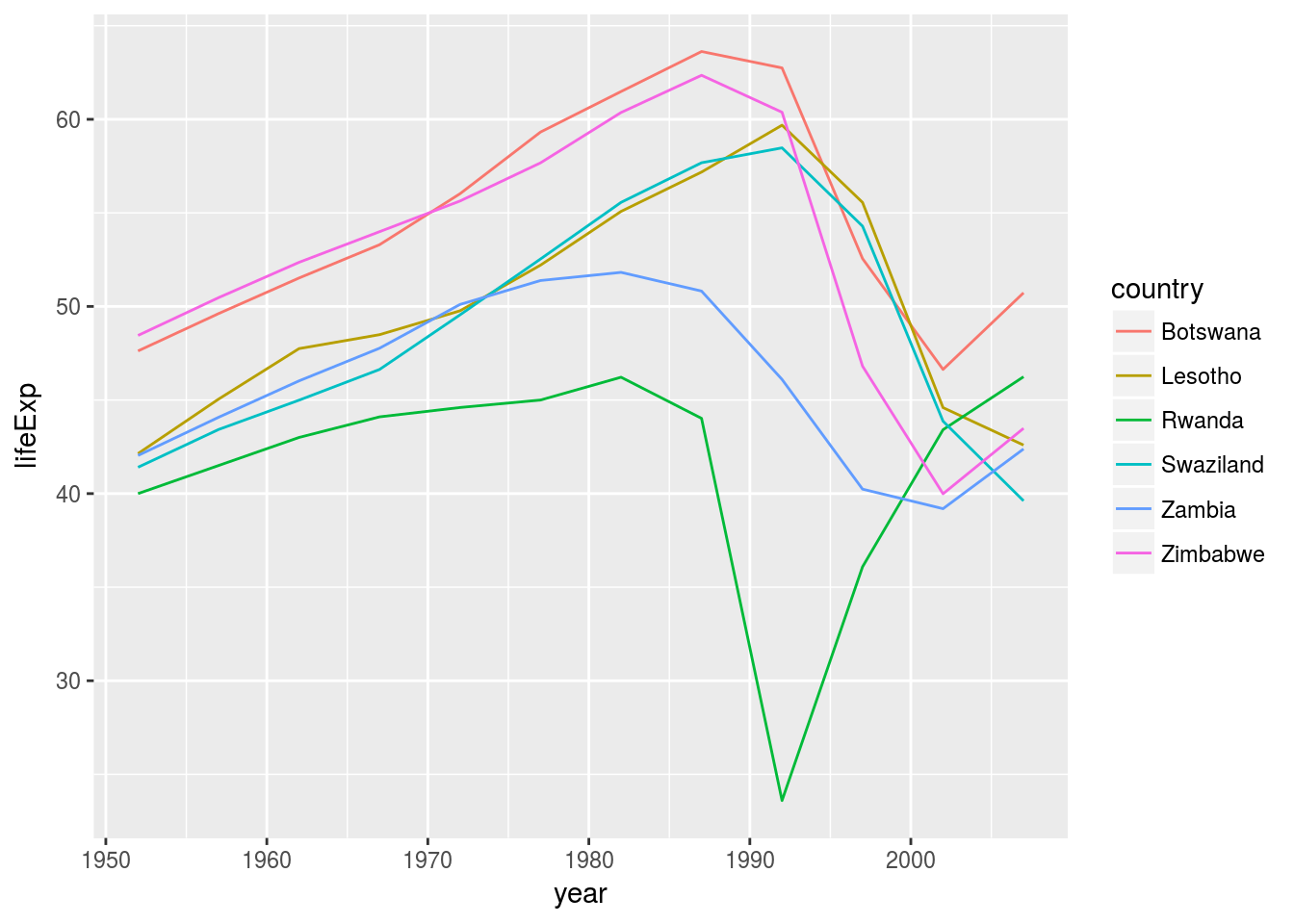### 25.2.4 25.2.5 Exercises

1. A linear trend seems to be slightly too simple for the overall trend. Can you do better with a quadratic polynomial? How can you interpret the coefficients of the quadratic? (Hint you might want to transform `year` so that it has mean zero.)
``````modquad <- function(df){
lm(data = df, lifeExp ~ poly(year,2))
}

mutate(year = year - mean(year)) %>%
group_by(country)%>%
nest()%>%

# data here is the column name, so is model.
)
``````## # A tibble: 1,704 x 7
##        country continent  year lifeExp      pop gdpPercap      resid
##         <fctr>    <fctr> <dbl>   <dbl>    <int>     <dbl>      <dbl>
##  1 Afghanistan      Asia -27.5  28.801  8425333  779.4453  0.6223132
##  2 Afghanistan      Asia -22.5  30.332  9240934  820.8530 -0.1662073
##  3 Afghanistan      Asia -17.5  31.997 10267083  853.1007 -0.6321523
##  4 Afghanistan      Asia -12.5  34.020 11537966  836.1971 -0.5515220
##  5 Afghanistan      Asia  -7.5  36.088 13079460  739.9811 -0.2373162
##  6 Afghanistan      Asia  -2.5  38.438 14880372  786.1134  0.5474650
##  7 Afghanistan      Asia   2.5  39.854 12881816  978.0114  0.5868217
##  8 Afghanistan      Asia   7.5  40.822 13867957  852.3959  0.3667537
##  9 Afghanistan      Asia  12.5  41.674 16317921  649.3414  0.2192612
## 10 Afghanistan      Asia  17.5  41.763 22227415  635.3414 -0.5026558
## # ... with 1,694 more rows``````

Check the residuals

``````unnest(quad, resids) %>%
ggplot(aes(group = country))+
geom_line(aes(x = year, y = resid))+
facet_wrap(~continent, nrow = 2)``````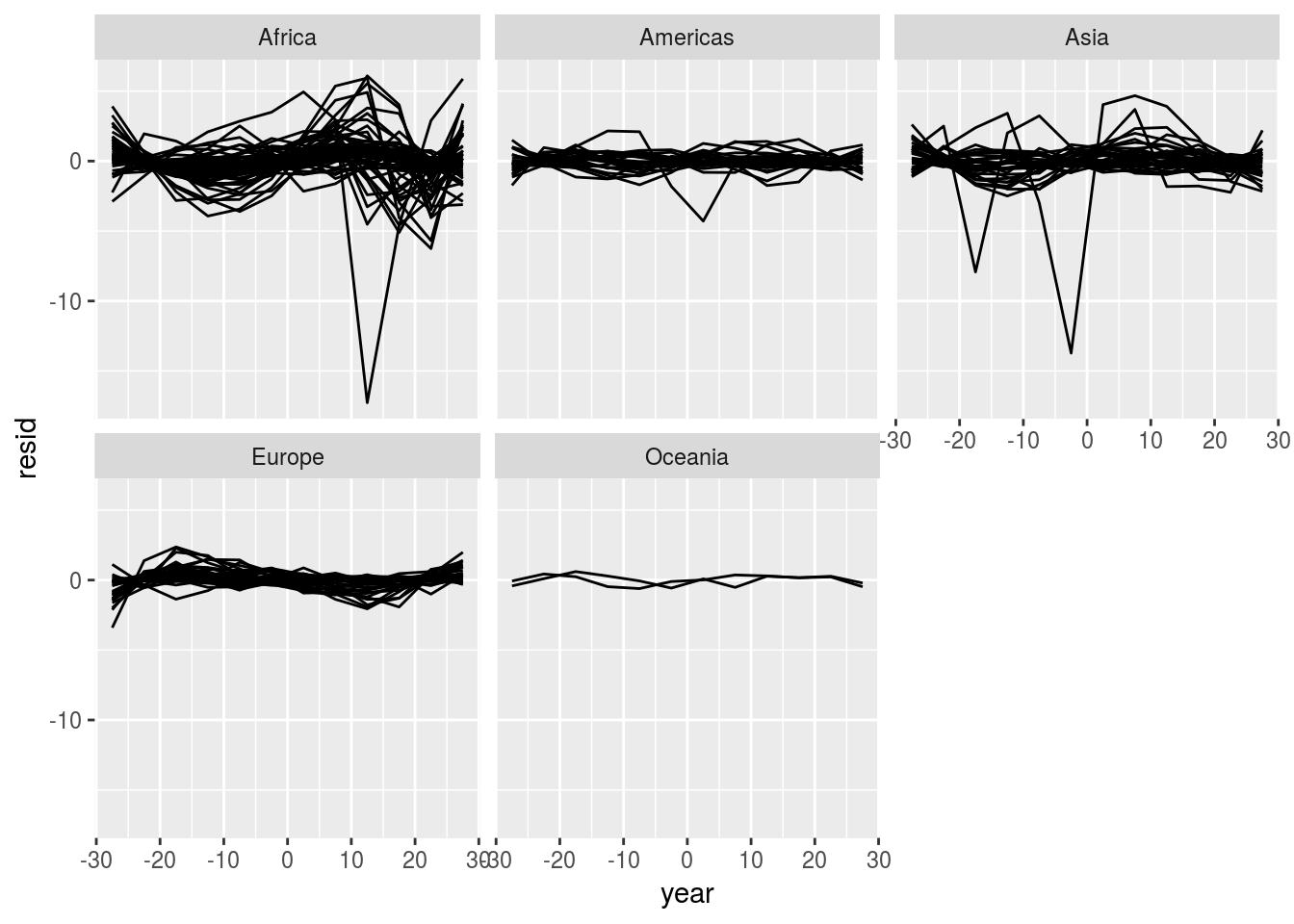Check the quality

``````quad %>%
mutate(glance = map(model, broom::glance))%>%
unnest(glance, .drop = TRUE)%>%
arrange(r.squared)``````
``````## # A tibble: 142 x 12
##             country  r.squared adj.r.squared    sigma   statistic
##              <fctr>      <dbl>         <dbl>    <dbl>       <dbl>
##  1           Rwanda 0.01735115    -0.2010153 6.912349  0.07945888
##  2          Liberia 0.61279138     0.5267450 1.664180  7.12164206
##  3           Uganda 0.64045980     0.5605620 2.484068  8.01598555
##  4         Cambodia 0.68205481     0.6114003 5.567185  9.65338275
##  5         Botswana 0.69396257     0.6259542 3.626425 10.20408358
##  6          Lesotho 0.70356998     0.6376966 3.559901 10.68064850
##  7         Zimbabwe 0.71095742     0.6467257 4.203267 11.06864030
##  8           Zambia 0.72850734     0.6681756 2.565257 12.07503373
##  9 Congo, Dem. Rep. 0.72952938     0.6694248 1.649671 12.13766664
## 10        Swaziland 0.73107550     0.6713145 3.762450 12.23332097
## # ... with 132 more rows, and 7 more variables: p.value <dbl>, df <int>,
## #   logLik <dbl>, AIC <dbl>, BIC <dbl>, deviance <dbl>, df.residual <int>``````

Compare to the linear model, the `r.squared` is much better.

``````quad %>%
mutate(glance = map(model, broom::glance))%>%
unnest(glance, .drop = TRUE) %>%
ggplot(aes(r.squared)) +
geom_histogram(bins = 100)``````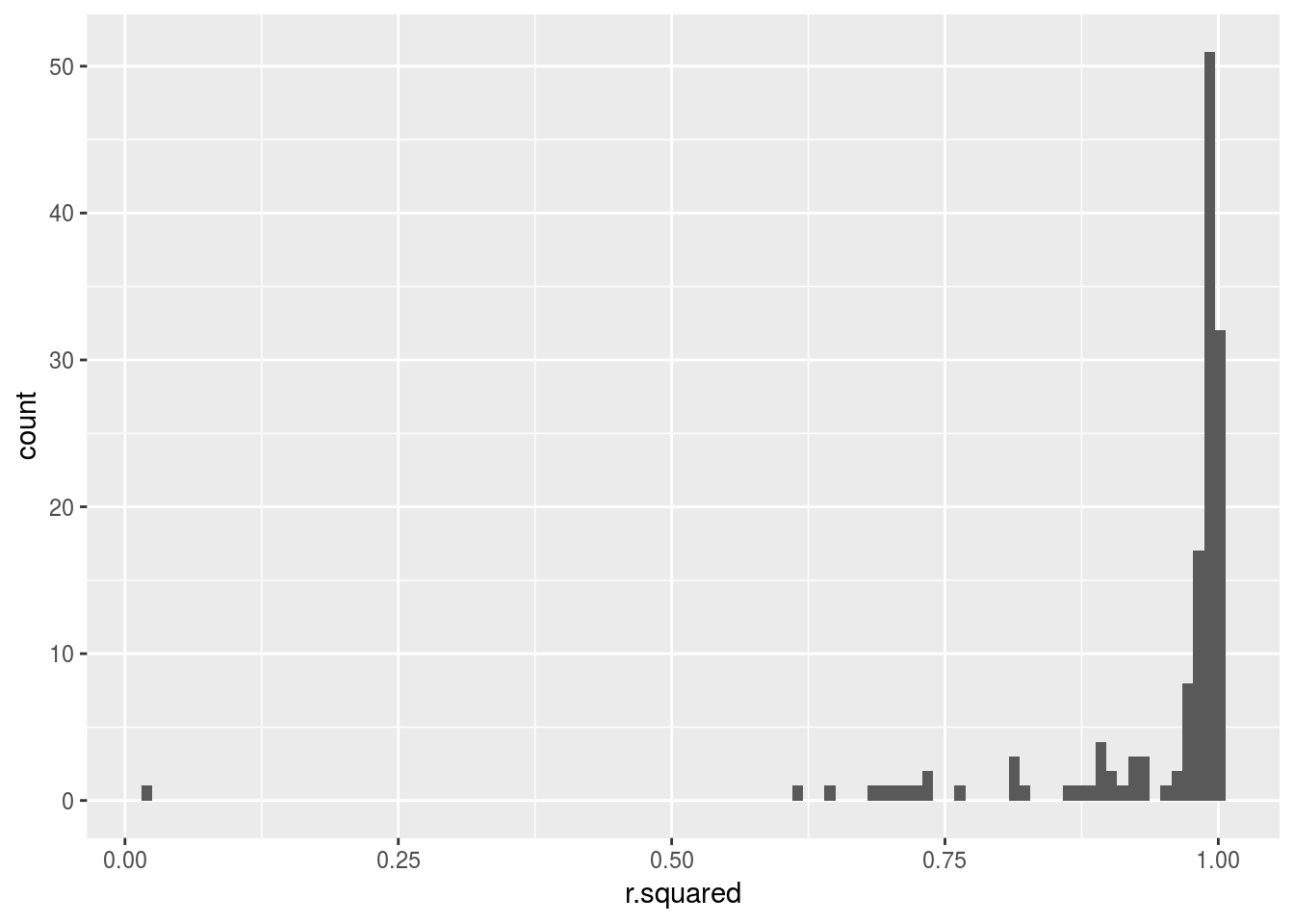1. Explore other methods for visualising the distribution of `R^2` per continent. You might want to try the `ggbeeswarm` package, which provides similar methods for avoiding overlaps as jitter, but uses deterministic methods.
``````library(ggbeeswarm)
gapminder %>%
mutate(year = year - mean(year)) %>%
group_by(continent,country)%>%
nest() %>%
mutate(glance = map(model, broom::glance))%>%
unnest(glance) %>%
ggplot(aes(x= continent, y =r.squared,color = continent)) +
geom_beeswarm()``````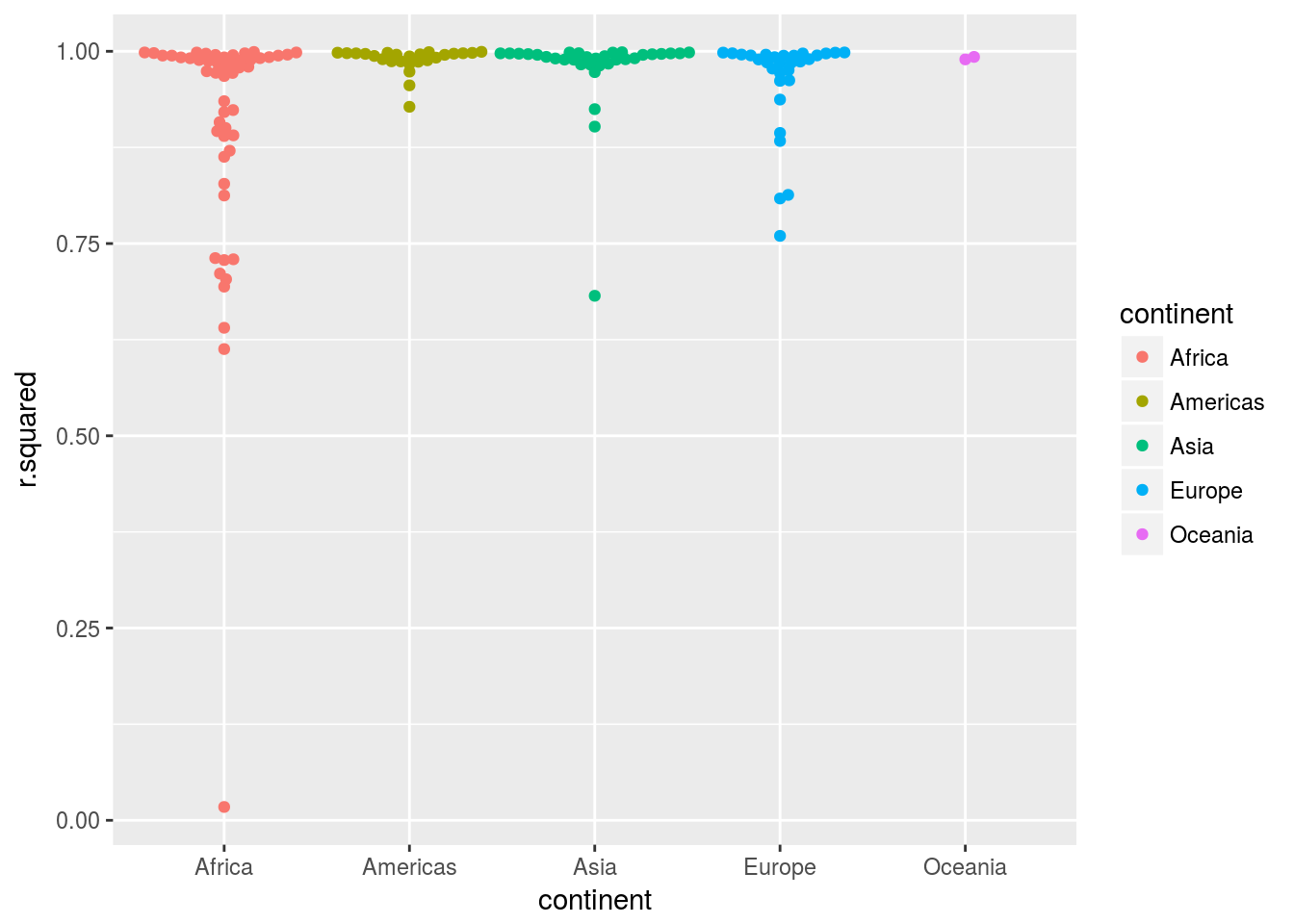It is clear that many Africa countries have patterns not captured by the polynomial functions.

3.To create the last plot (showing the data for the countries with the worst model fits), we needed two steps: we created a data frame with one row per country and then semi-joined it to the original dataset. It’s possible avoid this join if we use `nnest()` instead of `unnest(.drop = TRUE)`. How?

``````gapminder %>%
mutate(year = year - mean(year)) %>%
group_by(country)%>%
nest() %>%
mutate(glance = map(model, broom::glance))%>%
unnest(glance) %>%
unnest(data) %>%
semi_join(gapminder, by = c("pop","country")) %>%
arrange(r.squared) %>%
filter(r.squared %in% unique(r.squared)[1:6])%>%
ggplot(aes(x = year + mean(gapminder\$year), y = log(pop) )) +
geom_line(aes(color = country))``````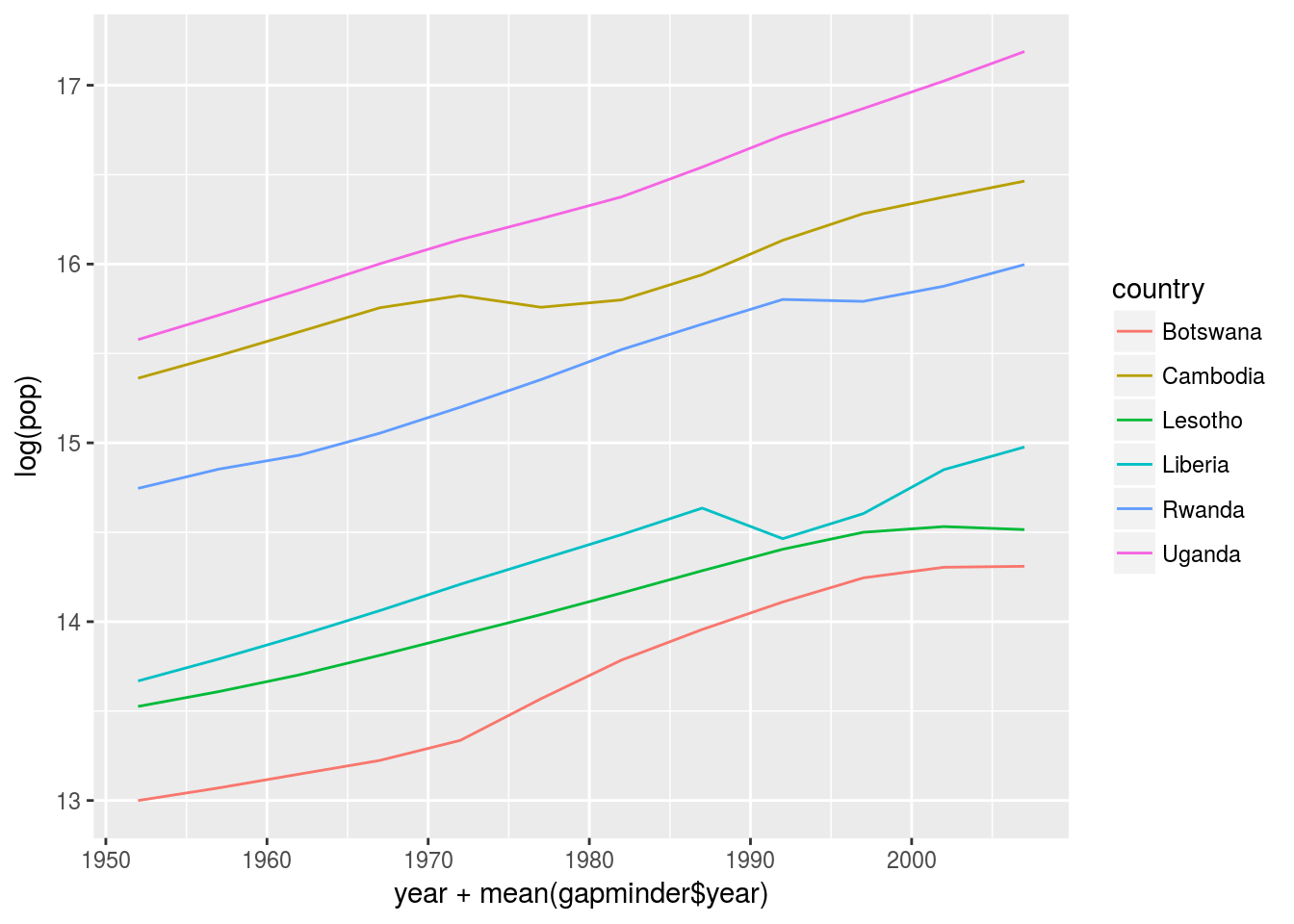## 25.3 List-columns

Note: The meaning of list-columns is a column as a list contains arbitary data structure you want.

``````# example of creating a list-columns
tibble(
x = list(1:3,3:5),
y = list(letters[1:4],as.factor(letters[5:9]))
)``````
``````## # A tibble: 2 x 2
##           x          y
##      <list>     <list>
## 1 <int >  <chr >
## 2 <int > <fctr >``````

## 25.4 Creating list-columns

Note: 1. `tidyr::nest()` to convert a `grouped` data frame into nested data frame. 2. `mutate()` with vectorised functions. 3. `summarise()` with summary functions

#### 25.4.0.2 From vectorised functions

``````df <- tribble(
~x1,
"a,b,c",
"d,e,f,g"
)
df %>%
mutate(x2 = stringr::str_split(x1, ","))``````
``````## # A tibble: 2 x 2
##        x1        x2
##     <chr>    <list>
## 1   a,b,c <chr >
## 2 d,e,f,g <chr >``````

`purrr::invoke_map` is a powerful function that you get a function and pass a list of parameters to it.

``````sim <- tribble(
~f,      ~params,
"runif", list(min = -1, max = -1),
"rnorm", list(sd = 5),
"rpois", list(lambda = 10)
)

sim %>%
mutate(sims = invoke_map(f, params, n = 10))``````
``````## # A tibble: 3 x 3
##       f     params       sims
##   <chr>     <list>     <list>
## 1 runif <list > <dbl >
## 2 rnorm <list > <dbl >
## 3 rpois <list > <int >``````
``````rm(list = ls())
sim <- tibble::tribble(
~f,      ~params,
"runif", list(min = -1, max = -1),
"rnorm", list(sd = 5),
"rpois", list(lambda = 10)
)%>%
mutate(sims = purrr::invoke_map(f, params, n = 10))``````
``````mydata <-
tibble(col_a = rep(c("a", "b"), 5)) %>%
mutate(col_b = map(col_a, function (x) { list(a = x, b = x, c = x) }))
mydata%>%
filter (col_a == "a")``````
``````## # A tibble: 5 x 2
##   col_a      col_b
##   <chr>     <list>
## 1     a <list >
## 2     a <list >
## 3     a <list >
## 4     a <list >
## 5     a <list >``````
``````mydata <-
tibble(col_a = rep(c("a", "b"), 5)) %>%
mutate(col_b = map(col_a, function (x) { list(a = x, b = x, c = x) }))%>%
filter (col_a == "a")
class(mydata)``````
``##  "tbl_df"     "tbl"        "data.frame"``

#### 25.4.0.3 From multivalued summaries

See `quantile()`

#### 25.4.0.4 From a named list

See `enframe()`

### 25.4.5 Exercises

1. List all the functions that you can think of that take a atomic vector and return a list.

`quantile` is an example

1. Brainstorm useful summary functions that, like `quantile()`, return multiple values.

You can write one for example as the example I constructed from answers based on this post

``````df <- data.frame(group=c('A','A','A','A','B','B','B','B','C','C','C','C'), x=rnorm(12,1,1), y=rnorm(12,1,1))
f <- function(x,y) list(setNames(coef(lm(x ~ y)), c("a", "b")))
df %>%
group_by(group)%>%
summarise(coef = f(x,y))``````
``````## # A tibble: 3 x 2
##    group      coef
##   <fctr>    <list>
## 1      A <dbl >
## 2      B <dbl >
## 3      C <dbl >``````
1. What’s missing in the following data frame? How does `quantile()` return that missing piece? Why isn’t that helpful here?

Not sure what the question is asking for.

1. What does this code do? Why might might it be useful?

I slightly modified the code to make its behavior more explicit

``````myfun <- function(x){
list(quantile(x))
}

mtcars %>%
group_by(cyl) %>%
summarise_each(funs(myfun))``````

This code apply the `list` function to each variable of the grouped data frames. This may be useful if you want to apply different `summarize` functions to different columns. Here we just use the same function `list`.

## 25.5 Simplifying list-columns

### 25.5.1 List to vector

``````df <- tribble(
~x,
letters[1:5],
1:3,
runif(5)
)``````

`purrr::map_char` takes a column whose element is a list and map each list to a `char` using provided function. Similar ideas apply for `map_int`, etc.

### 25.5.2 Unnest

unnesting two columns simultaneously requires they have exactly the same shape. The following code fails

``````df2 <- tribble(
~x, ~y,           ~z,
1, "a",         1:2,
2, c("b", "c"),   3
)
df2 %>% unnest(y, z)``````

### 25.5.3 Exercises

1. Why might the `lengths()` function be useful for creating atomic vector columns from list-columns?

Because you can’t use `count` directly and `lengths()` is equivalent to the `n()` when the data frame is not nested.

The following three ways all calculate the number of observables in each group.

``````df <- data.frame(group=c('A','A','A','A','B','B','B','B','C','C','C','C'), x=rnorm(12,1,1), y=rnorm(12,1,1))

df %>%
group_by(group)%>%
summarize(n = n())``````
``````## # A tibble: 3 x 2
##    group     n
##   <fctr> <int>
## 1      A     4
## 2      B     4
## 3      C     4``````
``````df %>%
group_by(group)%>%
nest() %>%
mutate(
n = map_int(data,nrow)
)``````
``````## # A tibble: 3 x 3
##    group             data     n
##   <fctr>           <list> <int>
## 1      A <tibble [4 x 2]>     4
## 2      B <tibble [4 x 2]>     4
## 3      C <tibble [4 x 2]>     4``````
``````df %>%
group_by(group) %>%
summarize_each(funs(list)) %>%
mutate(n = map_int(x,length))``````
``````## `summarise_each()` is deprecated.
## Use `summarise_all()`, `summarise_at()` or `summarise_if()` instead.
## To map `funs` over all variables, use `summarise_all()```````
``````## # A tibble: 3 x 4
##    group         x         y     n
##   <fctr>    <list>    <list> <int>
## 1      A <dbl > <dbl >     4
## 2      B <dbl > <dbl >     4
## 3      C <dbl > <dbl >     4``````
1. List the most common types of vector found in a data frame. What makes lists different?
1. bool
2. numeric
3. integer
4. factor
5. char List is special because it is nested, a list can contain other lists and any other kind of elements.

## 25.6 Making tidy data with broom

Note:

To turn models into tidy data frames

1. `broom::glance(model)`
2. `broom::tidy(mdoel)`
3. `broom::augment(model,data)`